# Fault diagnosis of wireless sensor network based on optimized probabilistic neural network

Fault diagnosis of wireless sensor network based on optimized probabilistic neural network

Haohai FuJun Xu Hua Zhang Min Zhang Xinxin Xu

School of Computer Technology and Engineering, Changchun Institute of Technology, Changchun 130012, China

Jilin Provincial Key Laboratory of Changbai Historical Culture and VR Reconstruction Technology, Changchun 130012, China

Changchun Institute of Technology, Changchun 130012, China

Corresponding Author Email:
sdtsfhh@163.com
Page:
295-308
|
DOI:
https://doi.org/10.3166/JESA.51.295-308
|
Accepted:
|
Published:
31 December 2018
| Citation

OPEN ACCESS

Abstract:

This paper proposes a node fault diagnosis algorithm for wireless sensor network (WSN) based on rough set and optimized probabilistic neural network (PNN). Specifically, the energy consumption of the diagnosis was reduced through rough set reduction of fault attributes. The attribute combinations were selected based on the correlation degree between attributes in the post-reduction attribute combination, rather than subjective judgement. Besides, an optimized PNN was adopted as the fault classification model. Through simulations, it is proved that the proposed algorithm can accurately and efficiently complete the task of WSN node fault diagnosis, especially when the sample contains lots of redundancy and noises. The research results shed new light on the fault diagnosis of WSN nodes and the application of artificial neural networks.

Keywords:

wireless sensor network (WSN), probabilistic neural network (PNN), fault diagnosis, rough set

1. Introduction

Wireless sensor network (WSN) is a self-organized network consisting of multiple low-cost sensor nodes, which are capable of data acquisition, processing and transmission. The network supports the real-time monitoring of the deployment environment, so that users can obtain necessary information anytime and anywhere (Hong et al., 2010). Thus, the WSN has been applied extensively in such fields as national defense, environment monitoring and health care (Li and Gao, 2008). Nevertheless, this network may suffer from dysfunction and even paralysis, owing to the complexity of the deployment environment and the defects of network nodes (e.g. proneness to damage and constraints on power, computing and storage capacity). Since most WSN faults are attributable to the fault of node modules, it is imperative to develop an effective and applicable fault diagnosis algorithm for these modules (Duh et al., 2013; Oh et al., 2012). Such an algorithm would extend the service time and ensure the stability of the entire network.

Most WSN fault diagnosis methods are founded on one of the following three bases: statistical principles, classification (Zhang et al., 2015) and spatial features of the acquired data (Akbari et al., 2011; Banerjee et al., 2011). Typical examples are neural networks (Obst, 2009) and fuzzy reasoning (Sarkis et al., 2012; Khan et al., 2012). These methods boast a good diagnostic effect but cannot locate mode modules other than sensors. To make up for the defect, a common strategy is to carry out node module diagnosis based on the correspondence between fault class and fault symptom of WSN nodes. By this method, a classification model can be established with fault symptoms as the attributes and fault classes as the outputs. Nevertheless, the diagnostic effect is still restrained by the redundant and irrelevant attributes in the fault sample. After all, there are always some noises in the data captured by the WSN, due to the defects of the network and the environment (Antoinesantoni et al., 2009). In addition to the diagnostic effect, the energy consumption is also negatively affected by these noises. Therefore, the reduction and elimination of noises are essential to the low energy consumption and high accuracy of the fault diagnosis.

Reference (Lin et al., 2007) carries out rough set reduction of attributes and performs fault diagnosis based on full matching of attributes. However, attribute combination after the reduction is too subjective and the diagnosis effect is poor when the data are not completely reliable. Based on rough set and Bayesian method, Reference (Pan et al., 2009) filters out the redundant information in the original data and diagnoses node fault effectively. Nonetheless, the attribute reduction combinations are selected randomly, rather than optimized, for fault classification, and the parameters of the classification model are too complicated. Reference (Li et al., 2009) carries out node fault diagnosis by naïve Bayes and attribute reduction based on independent component analysis (ICA). Nevertheless, the number of post-reduction attributes is greater than that of rough ensemble, and is easily affected by data reliability. In general, the existing diagnosis algorithm have many problems, including but not limited to high subjectivity, incomplete diagnosis attributes, random selection of post-reduction attributes, and complex parameters of classification model.

In view of the above, this paper proposes a node fault diagnosis algorithm for WSN based on rough set and optimized probabilistic neural network (PNN). Specifically, the energy consumption of the diagnosis was reduced through rough set reduction of fault attributes. The attribute combinations were selected based on the correlation degree between attributes in the post-reduction attribute combination, rather than subjective judgement. Besides, an optimized PNN was adopted as the fault classification model. The proposed method was proved effective and feasible through experimental analysis.

2. WSN node fault diagnosis model

2.1. WSN node fault classification

As shown in Figure 1, a WSN node consists of four indispensable modules: sensor, processor, wireless communication and power supply. These modules jointly perform the tasks of the node by completing their own duties. Since each module has its own features, the node faults can be classified easily module by module. In this way, fault positioning is equivalent to the identification of fault-prone modules. Following this train of thought (Ma et al., 2011; Chen et al., 2010), this paper divides node faults into power fault, processor fault, wireless communication fault and sensor fault, considering the structure of WSN nodes.

## 1.jpg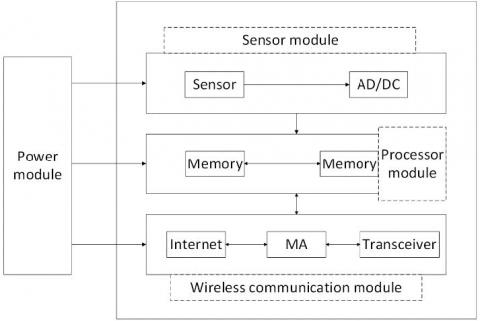Figure 1. Structure of a WSN node

2.2. Fault diagnosis model

In our fault diagnosis model, fault and its corresponding symptom are taken as samples. Each symptom corresponds to the feature attribute of a specific fault. The specific fault is a class attribute, which was used to construct the decision table. To eliminate redundancy, the original attributes of each sample was reduced by the improved discernable matrix, according to the theory of rough set reduction. This leads to multiple simplified attribute combinations. Bedsides, the dimension of the original feature space was reduced, and the noise interference in fault diagnosis was prevented. Then, the samples were reconstructed with the optimal attributes, and the PNN diagnosis model was established and optimized for fault diagnosis. The structure of the diagnosis model is illustrated in Figure 2.

## 2.jpg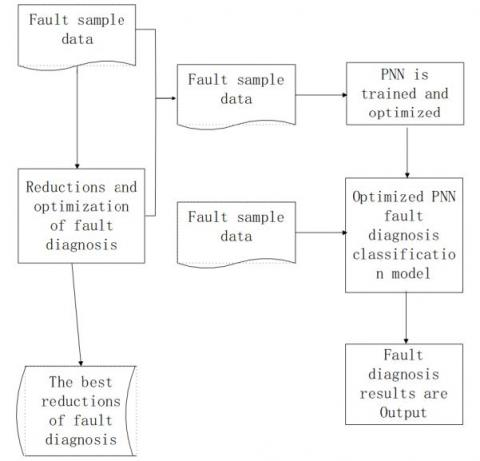Figure 2. Structure of fault diagnosis model

3. Attribute reduction and optimization

3.1. Attribute reduction

The attribute reduction was realized based on the rough set theory (Luo and Shao, 2006; Chen et al, 2007) - Les $=<U_{\mathrm{D}}, \mathrm{R}, \mathrm{V}, \mathrm{f}>$ be the decision table, where $\mathrm{S}$ is the knowledge expression system, $\mathrm{U}=\left\{\mathrm{x}_{1}, \mathrm{x}_{2}, \ldots \mathrm{x}_{\mathrm{m}}\right\}$ is a domain, $\mathrm{R}=\mathrm{P} \cup \mathrm{D}$ is the attribute set, $\mathrm{V}$ is the value range of $\mathrm{m}[\mathrm{i}]\left(\mathrm{x}_{\mathrm{j}}\right)\left(\mathrm{V}=\mathrm{U} \mathrm{V}_{\mathrm{B}}(\mathrm{m}[\mathrm{i}] \mathrm{E} \mathrm{R})\right)$ and $\mathrm{f} : \mathrm{U} \times \mathrm{R} \rightarrow \mathrm{V}$ is an information function. Note that the knowledge expression system corresponds to the WSN node fault diagnosis system; the P

and D  represent the condition attribute set and the decision attribute set, respectively, and the two sets correspond to fault  symptom set and the fault class fet, respectively; $\mathrm{m}[\mathrm{i}]\left(\mathrm{x}_{\mathrm{i}}\right)$ is the value of the sample $\mathrm{x}_{\mathrm{i}}$ on the attribute $\mathrm{m}[\mathrm{i}] .$ Each $\mathrm{m} \in \mathrm{R}, \mathrm{x} \in \mathrm{U},$ that satisfies $\mathrm{f}(\mathrm{x}, \mathrm{m}) \in \mathrm{V}_{\mathrm{R}} \cdot \mathrm{C}_{\mathrm{D}}(\mathrm{i}, \mathrm{j})$

is an element in the i-row and $\mathrm{j}$ -column recognizable matrix:

$C_{D}(i, j)=\left\{\begin{array}{l}{m[k], \mathrm{d}\left(x_{j}\right) \neq \mathrm{d}\left(x_{i}\right)} \\ {0 \quad, \mathrm{d}\left(x_{j}\right)=\mathrm{d}\left(x_{i}\right)}\end{array}\right.$           (1)

where

$\left\{\mathrm{m}[\mathrm{k}] | \mathrm{m}[\mathrm{k}] \in \mathrm{P} \wedge \mathrm{m}[\mathrm{k}]\left(\mathrm{x}_{\mathrm{i}}\right) \neq \mathrm{m}[\mathrm{k}]\left(\mathrm{x}_{\mathrm{j}}\right)\right\}$

Based on the improved discernible matrix, the attribute reduction can be realized through the following steps:

(1) By the above definitions, create $\mathrm{C}_{\mathrm{D}}(\mathrm{i}, \mathrm{j})$

against the fault sample decision table.

(2) Simplify the matrix: remove the set of single fault attribute elements from the matrix and set their positions to zero.

(3) When

$\mathrm{C}_{\mathrm{D}}(\mathrm{i}, \mathrm{j}) \neq 0$ and $\mathrm{C}_{\mathrm{D}}(\mathrm{i}, \mathrm{j}) \neq \emptyset$ , obtain Ti from row i of the matrix:

$T_{i}=V_{m[i] \in C_{D}(i, j)} m[i]$       (2)

(4) Find T through the conjunction of all Ti in (3):

$T=\Lambda_{C_{D}(i, j) \neq 0}, \quad c_{D}(i, j) \neq \emptyset T_{i}$          (3)

(5) Simplify the conjunctive result T and convert it into a disjunction method.

$T^{\prime}=V T$         (4)

(6) Add the single attribute in (2) to each conjunct. Each conjunctive term in disjunctive normal form T′ represents an attribute reduction.

3.2. Acquisition of optimal attributes

The post-reduction results are often selected by the least reduced number of attributes (Chang et al., 1999), the simplest rules (Zhang et al., 2005), or the largest reduction (Wang, 2003). However, none of these methods consider the correlation between attributes. Inspired by the principle of least interest in relational database design, this paper takes the correlation degree between attributes as the metric (Li et al., 2002), and selects the reduction result with the smallest mean correlation degree. The selection process is described as $\mathrm{S}=(\mathrm{U}, \mathrm{A}, \mathrm{V}, \mathrm{f}), \mathrm{a}, \mathrm{b} \in \mathrm{A}$

. The correlation between attributes  and  can be expressed as:

$r(a, b)=\frac{\operatorname{card}\left(\operatorname{Pos}_{a}(b) \cup \operatorname{Pos}_{b}(a)\right)}{\operatorname{card}(U)}$        (5)

where $\operatorname{card}(*)$ is the number of elements. It is clear that $r(a, b)$ is symmetric about $a$ and $b,$ and belongs to the range $[0,1]$ . The value of $r(a, b)$ is positively correlated $d$ to the correlation degree between $a$ and $b .$ In other words, the size of $r(a, b)$ characterizes how closely the attributes $a, b$ each divide the equivalence classes.

4. Fault diagnosis based on optimized PNN

4.1. Fault diagnosis model based on PNN

Proposed by Specht (Specht, 1990), the PNN is a neural network model capable of statistical classification.  The simple model is fast in learning and convenient for sample addition. The class density can be obtained by kernel density estimation (Evagorou et al., 2007), laying the basis for the classification of samples. The samples are classified by Bayesian minimum risk criteria, with full use of prior knowledge. However complex the problem is, the optimal classification solution can always be obtained. The PNN fault classifier can complete the fault diagnosis based on Bayesian decision theory and kernel density estimation.

Let $\mathrm{P}=\left\{\mathrm{A}_{1}, \mathrm{A}_{2} \cdots \mathrm{A}_{\mathrm{n}}\right\}$

be the set of post-reduction fault attributes, with  n being the dimension of sample data attribute; Let $D=\left\{\mathrm{d}_{1}, \mathrm{d}_{2} \cdots \mathrm{d}_{\mathrm{m}}\right\}$  be the set of fault class, with  being the number of classes; Let P be the reduced fault attribute for diagnosis. Then, reconstruct the fault sample decision table $\mathrm{X}=\left\{\mathrm{x}_{1}, \mathrm{x}_{2} \cdots \mathrm{x}_{\mathrm{i}}\right\}$ , where $\mathrm{X}_{\mathrm{i}}=\left\{\mathrm{a}_{1}, \mathrm{a}_{2} \cdots \mathrm{a}_{\mathrm{n}}, \mathrm{d}_{\mathrm{j}}\right\}$  is the single fault information. The terms $a_{1}, a_{2} \cdots a_{n}$  represent fault symptoms, whose values correspond to$A_{i} ;$ the term $d_{j}$ represent the fault class.

For the sample data X, the input layer firstly receives the data and then transfers it to the pattern layer. Then, the pattern layer and the summation layer compute the class density of x by the following formula:

$P\left(x | d_{i}\right)=\frac{1}{N_{i}} \sum_{k=1}^{N_{i}} \frac{1}{(2 \pi)^{l / 2 \sigma l}} \exp \left[\frac{-\left(x-x_{i j}\right)^{\mathrm{T}}\left(x-x_{i j}\right)}{2 \sigma^{2}}\right]$         (6)

where $i=1,2, \cdots M,$ with $M$ being the total number of training samples; $j=1,2 \cdots N ;$ $N_{i}$ is the number of samples in class $i,$ i.e. the number of hidden neurons in the $i$ -th pattern of the PNN; $l$ is the dimension of the sample space; $\sigma$ is the smooth factor; $x_{i j}$ is the $j$ -th hidden center vector of the $i$ -th pattern.

Finally, the output layer exports the fault class corresponding to the maximum posterior probability by the following formula:

$\rho(x)=\arg \max \left\{\alpha_{d i} P\left(x | d_{i}\right)\right\}$           (7)

where $\alpha_{\mathrm{di}}$ is the prior probability of $\mathrm{d}_{\mathrm{i}}$.

4.2. Optimization of fault diagnosis model

The selection of the smooth factor σ directly bears on the classification effect of the PNN. At present, there is no effective method to estimate the smooth factor. Most existing approaches are empirical in nature (Specht, 1996). What is worse, the smooth factor has a limited effect when the sample size is rather small. To ensure the effect of fault diagnosis, the particle swarm optimization (PSO) (Wu et al., 2006) is introduced to optimize the selection of PNN smooth factor σ, and thus enhance the classification effect. In the PSO, the accuracy of fault diagnosis is set as the fitness function:

$F i t=\frac{F C_{p}}{F C_{r}} \times 100 \%$          (8)

where $\mathrm{FC}_{\mathrm{p}}$ is the number of faults diagnosed by the model; $\mathrm{FC}_{\mathrm{r}}$ is the actual number of faults.

The PSO optimization involves the following steps:

(1) Input the feature data and class of the fault sample, and output the classification data.

(2) Initialize the particle group and configure the initial parameters (e.g. number of particles, inertia weight and learning factor).

(3) Set the optimal fitness and optimal position.

(4) Initialize the network, map each particle as a PNN smooth factor, and construct the PNN.

(5) Train and test the network.

(6) Compare the fitness of each particle and that of individual extreme point, and determine whether the update is necessary.

(7) Determine if the termination condition is satisfied. If yes, terminate the iteration; otherwise, update the speed and position of the particles and return to (3).

The optimal solution for the mapped particles is the smooth factor. Then, the PNN should be inputted to complete the construction of the optimized fault diagnosis model.

5. Simulation and analysis

For the WSN node, the correspondence between fault symptoms and attributes is shown in Table 1, and that between fault symptoms and fault classes are shown in Table 2. According to the two tables, the fault sample decision table was created and recorded in Table 3. For the space limit, only a few fault samples are listed. However, the actual diagnosis uses all fault samples. The feedbacks on node faults were taken as the original data for simulation. The entire process involves attribute reduction, selection of optimal attribute, optimization of PNN and fault diagnosis.

Table 1. Correspondence between fault symptoms and attributes

 No. Type of symptoms Attribute value C Did the node respond to the inquiry command? C=1,No C=2,Yes C Did the node return a signal? C=1,No C=2,Yes C Did the node effectively execute the command from the sink node? C=1,No C=2,Yes C Is the temperature returned by the node is 0? C=1, Yes C=2, No C Is the node temperature far from the mean value? C=1, Yes C=2, No C Can the node transfer data to other nodes? C=1,No C=2,Yes C Is the node temperature higher than normal? C=1, Yes C=2, No C Is there a response to the change of the transmission frequency of the test node? C=1,No C=2,Yes

The initial table of node fault sample decision was taken as sample information. Then, the discernible matrix $C_{D}$ was established according to the definition of

discernable matrix. Using the attribute reduction algorithm, the minimum attribute reduction combinations $R_{1}=\{c, c, c, c\}$ and $R_{2}=\{c, c, c, c\}$ $\text { of the decision system were obtained (Table } 3) .$ According to the formula in Section $3.2, r\left(R_{1}\right)=1$ and $r\left(R_{2}\right)=0.8125$ were derived, indicating that $r\left(R_{1}\right)$ was greater $\operatorname{than} r\left(R_{2}\right) .$.

Table 2. Correspondence between fault symptoms and fault classes

 No. Fault class Corresponding symptom $d_{1}$ No fault No fault symptom $d_{2}$ Power fault C, C, C,C, C, C, C,C $d_{3}$ Sensor fault C, C,C $d_{4}$ Processor fault C, C, C,C $d_{5}$ Wireless communication fault C, C, C,C, C

Table 3. WSN node fault sample decision table

 U c c c c c c c c D 1 2 2 2 2 2 2 2 2 $d_{1}$ 2 1 2 1 2 1 1 2 2 $d_{1}$ 3 1 2 1 2 1 1 1 1 $d_{5}$ 4 1 2 1 2 2 1 2 1 $d_{5}$ 5 1 1 1 1 1 1 1 1 $d_{2}$ 6 1 1 1 1 1 1 1 1 $d_{2}$ 7 2 2 1 2 2 2 2 2 $d_{4}$ 8 2 2 1 2 2 2 2 2 $d_{4}$ 9 2 2 2 1 1 2 2 2 $d_{3}$ 10 2 2 2 2 1 2 2 2 $d_{3}$ 11 2 2 2 2 2 2 2 2 $d_{1}$ 12 1 2 1 2 1 1 2 2 $d_{1}$ 13 2 2 1 2 2 2 2 2 $d_{4}$ 14 2 2 1 2 2 2 2 2 $d_{4}$ 15 2 2 2 1 1 2 2 2 $d_{3}$ 16 2 2 2 2 1 2 2 2 $d_{3}$ 17 1 2 1 2 1 1 1 1 $d_{5}$ 18 1 2 1 2 2 1 2 1 $d_{5}$ 19 1 1 1 1 1 1 1 1 $d_{2}$ 20 1 1 1 1 1 1 1 1 $d_{2}$

According to the rule of merit, $\mathrm{R}_{2}=\{\mathrm{c}, \mathrm{c}, \mathrm{c}, \mathrm{c}\}$  was identified as the optimal reduction. The fault sample complex table (Table 4) was reconstructed based on the optimal reduction $\mathrm{R}_{2}$ .

As shown in the reconstructed sample decision complex table, the optimal PNN input was $R_{2}=\{c, c, c, c\}$ . Then, the fault diagnosis process was simulated in Matlab. Two combinations of reduction attribute $R_{1}=\{c, c, c, c\}$  and $R_{2}=\{c, c, c, c\}$  were determined as the attributes for fault diagnosis, fault samples were reconstructed and the optimized diagnosis model was set up. The diagnosis results are depicted in Figure 3, where the two bars stand for the two different combinations of reduction attributes.

As shown in the figure, the optimal attribute combination $R_{2}$  slightly outperformed $R_{1}$  in terms of diagnosis accuracy. The result validates the merit metric formula in Section 3.2. The use of the optimal combination overcomes the subjectivity and randomness in References (Pan et al., 2009; Li et al., 2009) and enhances the fault diagnosis effect.

Table 4. WSN node fault sample complex table

 U c c c c D 1 2 2 2 2 $d_{1}$ 2 1 2 1 2 $d_{1}$ 3 1 2 1 1 $d_{5}$ 4 1 2 2 1 $d_{5}$ 5 1 1 1 1 $d_{2}$ 6 1 1 1 1 $d_{2}$ 7 1 2 2 2 $d_{4}$ 8 1 2 2 2 $d_{4}$ 9 2 1 1 2 $d_{3}$ 10 2 2 1 2 $d_{3}$ 11 2 2 2 2 $d_{1}$ 12 1 2 1 2 $d_{1}$ 13 1 2 2 2 $d_{4}$ 14 1 2 2 2 $d_{4}$ 15 2 1 1 2 $d_{3}$ 16 2 2 1 2 $d_{3}$ 17 1 2 1 1 $d_{5}$ 18 1 2 2 1 $d_{5}$ 19 1 1 1 1 $d_{2}$ 20 1 1 1 1 $d_{2}$

## 3.jpg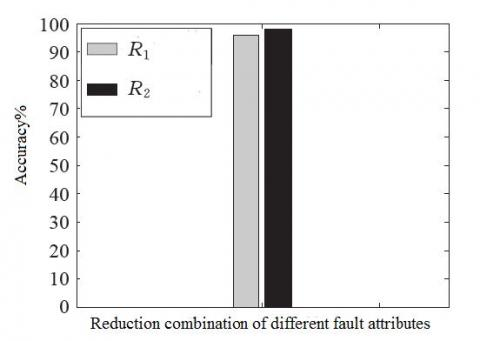Figure 3. Effects of different attribute combinations

To verify the effect of the optimized PNN fault diagnosis model, the model was simulated with optimal and non-optimal smooth factors, respectively. The results of the two scenarios are compared in Figure 4, where the two blocks illustrate the results before and after the optimization, respectively. The results show that the optimized PNN model achieved a 4.5% more accuracy result than the non-optimized model.

## 4.jpg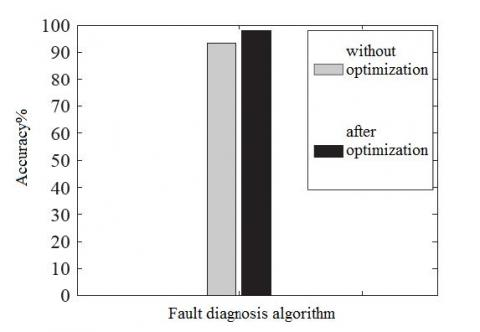Figure 4. Results before and after optimization

Finally, the proposed algorithm was contrasted with existing algorithms in References (Pan et al., 2009; Li et al., 2009; Ma et al., 2011). The simulation was conducted at different levels of data reliability, considering that the WSN data transmission is prone to external interference. The comparison results are shown in Figure 5 and Figure 6.

## 5.jpg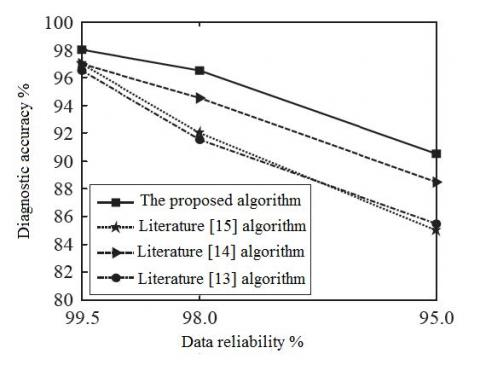Figure 5. Diagnosis accuracy of different algorithms

## 6.jpgFigure 6. Decrement of diagnosis accuracy of different algorithms

From Figures 5 and 6, it is clear that the proposed algorithm slightly outperformed the contrastive algorithms at the data reliability of 99.5%. With the decrease in data reliability, the accuracy of all algorithms exhibited a decreasing trend. However, the accuracy of the proposed algorithm remained at a high level and was less affected than that of the other algorithms. The good performance is attributable to the selection of attribute with the smallest correlation as the diagnosis attribute, rather than random selection. In this way, our algorithm reduces the impact of attribute correlation on fault diagnosis. Besides, the diagnosis effect is further improved by PNN optimization (Figure 3).

By contrast, the algorithm of Reference uses the exact match of attributes after reduction. This strategy works well at high data reliability, but becomes less effective when the data are not so reliable. The algorithm of Reference yields biased diagnosis due to the subjectivity of some parameters. As for the algorithm of Reference, the diagnosis effect decreased with the data reliability, because the number of post-reduction attributes is greater than that of rough ensemble.

In general, the proposed algorithm manages to remove the redundancy of diagnosis attributes through attribute reduction, reduce the computing load and avoid misdiagnosis caused by redundant attributes. The algorithm works particularly well at a low level of data reliability. The diagnosis effect can be further enhanced through optimized PNN classification.

6. Conclusions

This paper proposes a node fault diagnosis algorithm for WSN based on rough set and optimized PNN. Specifically, the energy consumption of the diagnosis was reduced through rough set reduction of fault attributes. The attribute combinations were selected based on the correlation degree between attributes in the post-reduction attribute combination, rather than subjective judgement. Besides, an optimized PNN was adopted as the fault classification model. Through simulations, it is proved that the proposed algorithm can accurately and efficiently complete the task of WSN node fault diagnosis, especially when the sample contains lots of redundancy and noises. The research results shed new light on the fault diagnosis of WSN nodes and the application of artificial neural networks.

Acknowledgment

This research was jointly supported by the Foundation of Jilin Province Education Department (JJKH20180985KJ) and Foundation of Jilin Provincial Science & Technology Department (20180622006JC, 20170101009JC).

References

Akbari A., Dana A., Khademzadeh A. (2011). Fault detection and recovery in wireless sensor network using clustering. International Journal of Wireless & Mobile Networks, Vol. 3, No. 1, pp. 130-138.

Antoinesantoni T., Santucci J. F., Gentili E. D. (2009). Performance of a protected wireless sensor network in a fire. Analysis of fire spread and data transmission. Sensors, Vol. 9, No. 8, pp. 5878-5886. https://doi.org/10.3390/s90805878

Banerjee I., Chanak P., Sikdar B. K. (2011). DFDNM: A distributed fault detection and node management scheme for wireless sensor network. Communications in Computer & Information Science, Vol. 192, pp. 68-81. https://doi.org/10.1007/978-3-642-22720-2_7

Chang L. Y., Wang G. Y., Wu Y. (1999). An approach for attribute reduction and rule generation based on rough set theory. Journal of Software, Vol. 10, No. 11, pp. 1206-1211.

Chen D., Wang C., Hu Q. (2007). A new approach to attribute reduction of consistent and inconsistent covering decision systems with covering rough sets. Information Sciences, Vol. 177, No. 17, pp. 3500-3518. https://doi.org/10.1016/j.ins.2007.02.041

Chen Y. J., Yuan S. F., Wu J. (2010). Research progress of fault diagnosis and fault-tolerant control in wireless sensor networks. Transducer and Microsystem Technologies, Vol. 29, No. 1 pp. 1-5. https://doi.org/10.3724/SP.J.1187.2010.00953

Duh D. R., Li S. P., Cheng V. W. (2013). Distributed fault-tolerant event region detection of wireless sensor networks. International Journal of Distributed Sensor Networks, Vol. 3, pp. 286-291.

Evagorou D., Kyprianou A., Lewin P. L. (2007). Classification of partial discharge signals using probabilistic neural network. IEEE International Conference on Solid Dielectrics, Vol. 7, pp. 609-615. https://doi.org/10.1109/ICSD.2007.4290887

Hong F., Chu H. W., Jin Z. K. (2010). Review of recent progress on wireless sensor network applications. Journal of Computer Research and Development, Vol. 47, No. s2, pp. 81-87.

Khan S. A., Daachi B., Djouani K. (2012). Application of fuzzy inference systems to detection of faults in wireless sensor networks. Neurocomputing, Vol. 94, No. 10, pp. 111-120. https://doi.org/10.1016/j.neucom.2012.04.002

Li J. Z., Gao H. (2008). Survey on sensor network research. Journal of Computer Research and Development, Vol. 45, No. 3 pp. 1-15.

Li K., Liu Y. S., Wang L. (2002). An approach for attribute reduction based on rough set theory. Computer Engineering and Applications, Vol. 38, No. 5, pp. 15-16.

Li Z. N., Fan T., Liu L. Z. (2009). Blind separation of the mechanical fault sources based on variational Bayesian theory. Journal of Vibration & Shock, Vol. 28, No. 6, pp. 12-16. https://doi.org/10.1109/MILCOM.2009.5379889

Lin L., Dai C. L., Wang H. J. (2007). Rough set theory based fault diagnosis of node in wireless sensor network. Journal of Beijing University of Posts & Telecommunications, Vol. 30, No. 4, pp. 69-73. https://doi.org/10.1631/jzus.2007.A1596

Luo X., Shao H. (2006). Developing soft sensors using hybrid soft computing methodology: A neurofuzzy system based on rough set theory and genetic algorithms. Soft Computing, Vol. 10, No. 1, pp. 54-60. https://doi.org/10.1007/s00500-005-0465-0

Ma C., Liu H. W., Zuo C. D. (2011). Hierarchical fault models for wireless sensor networks. Journal of Tsinghua University, Vol. 51, No. S1, pp. 1418-1423.

Obst O. (2009). Distributed fault detection in sensor networks using a recurrent neural network. Neural Processing Letters, Vol. 40, No. 3, pp. 261-273. https://doi.org/10.1007/s11063-013-9327-4

Oh S. H., Hong C. O., Choi Y. H. (2012). A malicious and malfunctioning node detection scheme for wireless sensor networks. Wireless Sensor Network, Vol. 4, No. 3, pp. 84-90.

Pan J. L., Ye X. H., Wang H. X. (2009). Node fault diagnosis in WSN based on the rough set and bays decision making. Chinese Journal of Sensors and Actuators, Vol. 22, No. 5, pp. 735-738.

Sarkis M., Hamdan D., Hassan B. E. (2012). Online data fault detection in wireless sensor networks. 2nd International Conference on Advances in Computational Tools for Engineering Applications (ACTEA), Beirut, pp. 61-65. https://doi.org/10.1007/978-3-642-16584-9_68

Specht D. F. (1996). Probabilistic neural network and general regression neural network. Fuzzy Logic and Neural Network Handbook, pp. 301-344. https://doi.org/10.1109/ICIP.1994.413718

Specht D. F. (1990). Probability neural networks. Neural Networks, Vol. 3, pp. 109-118.

Wang G. Y. (2003). Calculation methods for core attributes of decision table. Chinese Journal of Computers, Vol. 26, No. 5, pp. 611-615. https://doi.org/10.1023/A:1021351800842

Wu L. H., Wang Y. N., Yuan X. F., Liu Z. R. (2006). Research and application of compound optimum model particle swarm optimization. Systems Engineering & Electronics, Vol. 28, No. 28, pp. 1087-1090. https://doi.org/10.1360/jos172537

Zhang J., Wang J. M., He H. C. (2005). A Novel feature reduct algorithm based on feature correlation. Computer Engineering and Applications, Vol. 41, No. 28, pp. 55-57.

Zhang W., Han G., Feng Y. (2015). A novel method for node fault detection based on clustering in industrial wireless sensor networks. International Journal of Distributed Sensor Networks, Vol. 2, pp. 94-99. https://doi.org/10.1155/2015/230521